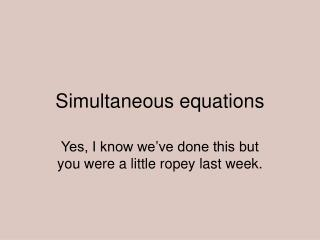DownloadDownload PresentationSimultaneous equations

# Simultaneous equations

Télécharger la présentation## Simultaneous equations

- - - - - - - - - - - - - - - - - - - - - - - - - - - E N D - - - - - - - - - - - - - - - - - - - - - - - - - - -
##### Presentation Transcript

1. Simultaneous equations Yes, I know we’ve done this but you were a little ropey last week.

2. 4 ways of solving them

3. Pros If you guess well, then easy to solve. Cons Hard to show your working. Only works for really simple ones. Can take a lot of time. 1 Guessing the answers.

4. Pros Works for all but the most complicated equations( A – level). Will give exact answer. Cons Need to show all working and work carefully. May need to multiply equations first 2 By adding/subtracting equations(traditional method)

5. Pros Will work every time Works for families of equations Cons Not always accurate Time consuming Long-winded, lots of room for mistakes to creep in. 3 Graphically

6. Pros Can be quickest way Best way for complicated equations e.g.. powers Cons Not suitable for all equations More likely you are looking at A – level paper. 4 Substitution